# What-If Analysis in ExcelArticle byJeevan A Y## What Is What-If Analysis In Excel?

The What-If Analysis is a feature used to observe the different scenarios when one or more variables change resulting in a change in the overall outcome. It helps us build different scenarios, achieve goal seek numbers, and create data tables using variables.

For instance, a printing shop prints approximately 1000 copies per day. Therefore, the estimated profit is calculated as \$500. So, now we need to know what the profit will be using what-if scenarios such as what if the company prints 1500 copies, 2000 copies, and 2500 copies. By using What-If analysis, we can create scenarios for profit.

So, let us learn how to use what if analysis in Excel in detail.

###### Key Takeaways
• What-If Analysis helps in creating and evaluating different scenarios. Thus, it helps users make the best selection from the available alternatives.
• Based on the requirement, we can choose the three methods: Scenario Manager, Goal Seek, and Data Table.
• Goal Seek changes only a single variable, so it is used to set only one cell value.
• Data Table is used to choose the best outcome when changing two variables.
• The scenario manager uses multiple variables simultaneously and returns output with different scenarios.

### Types Of What-if Analysis In Excel

There are three types of what-if analysis in excel. They are:

1. Scenario Manager
2. Goal Seek
3. Data Table

We need to choose any of the above three methods to perform a What-If analysis in excel.

So, let us learn about the three types in detail.

#### #1 – Scenario Manager

What is it?

Using Scenario Manager, we can make changes to multiple variables. For each change, we can create scenarios and compare one against another. Below are some of the key features of Scenario Manager.

• We can create different scenarios every time there is a change in a particular variable or multiple variables.
• We can create up to 32 scenarios.
• We can create a summary of all the scenarios by combining all the scenarios in the worksheet.
• We can give names to each scenario as per user requirements.

Step by Step Example

For instance, Mr. Peter is running an advertising company, and his revenue, cost, and profit details are shown below:

Mr. Peter knows that the more he pushes on indirect revenue, the more indirect cost increases. So now, he wants to know what would be his profit numbers when indirect revenue & indirect cost increase.

Mr. Peter estimates the following scenarios:

• When the Indirect Revenue is \$10,000, the Indirect Cost increases to \$8,000.
• When the Indirect Revenue is \$12,000, the Indirect Cost increases to \$9,000.
• When the Indirect Revenue is \$15,000, the Indirect Cost increases to \$14,000.

So, Mr. Peter wants to know which estimate will be best for his business and how his profit will be impacted when these scenarios occur.

Let us make use of scenario managers to create different profit scenarios.

The steps used to obtain results using what-if analysis in Excel with Scenario Manager are as follows:

Step 1: Go to the Data and click on the What-If Analysis from the Forecast group.

From the drop-down list, select the Scenario Manager.

Step 2: Now, we can see Scenario Manager window as shown below.

Step 3: Type the scenario name as Option 1.

Step 4: In the Changing Cells box, we need to choose the cells we like to change.

In this example, we want to change the Indirect Revenue (cell B3) and the Indirect Cost (cell B7).

So, choose cells B3 and B7.

Step 5: Click OK.

The Scenario Values window appears. Change the values of cells B3 & B7, respectively.

It is Mr. Peter’s first option or the scenario.

Step 6: Click on Add option since we need to create two more scenarios.

As soon as we click on Add, the Scenario Manager window pops up again. Now, let us type the name as Option 2.

Step 7: Since we want to change the Indirect Revenue (cell B3) and the Indirect Cost (cell B7), the cell references in Changing cells: box remain the same.

Step 8: Click on Add and change the B3 & B7 cell values.

Step 9: Similarly, click on Add and create the third scenario as Option 3.

Step 10: Click OK and change the cell values B3 & B7.

Step 11: Now, do not click on Add because we have created all the scenarios. Instead, select OK, and we will have a list of all the scenarios we have created so far.

Step 12: Click on Summary. The Scenario Summary window pops up. Here, we should choose the Result cells: i.e., the cell where we want to display the results.

Let us choose cell B10 (the Total Profit Cell) in our example.

Step 13: Click OK, and we will have a summary report of each scenario as highlighted in the below image:

We can see that the feature has provided the results with current values and the three scenarios we have created.

Interpretation of Scenarios: From the table, it is clear that Option 2 yields the maximum profit of \$23,000. So, Mr. Peter can choose Option 2.

If we look at Option 3, the indirect revenue is more, but due to an increase in the indirect cost, the profit comes down to \$21,000.

Likewise, we can create business scenarios and decide on the best-suited scenario using what if analysis in Excel.

#### #2 – Goal Seek

What is it?

Goal seek helps us know the required number to meet the targeted value. For instance, assume a student has completed five exams, and his anticipated total score is 400 out of 500, i.e., an average score of 80 per subject. However, he still has to write one more subject. Therefore, his target is to score more than the average score of 80. In this case, we can use Goal Seek. It helps him know the required score to achieve his targets.

Remember, while using goal seek, we can change only one variable.

Step by Step Example

For instance, Ms. Paula is a cricketer and has a target of getting at least 50 runs in the tournament. Out of 8, she played 7 matches and already scored 326 runs.

The below table shows the runs scored by Ms. Paula.

In cell B10, we have applied the AVERAGE Excel function, and the average obtained from matches is 47.

So, now in the tournament’s final match, she wants to know what the targeted score (goal) will be.

Therefore, we can use the Goal Seek feature to find the required score.

The steps used to obtain results using what-if analysis in Excel with Goal seek method are as follows:

Step 1: Go to the Data tab and click on the What-If Analysis option from the Forecast group.

Then, select the Goal Seek Excel option from the drop-down list.

Step 2: The Goal Seek window pops up.

Step 3: In the Set cell: box, we need to choose the cell references for which we need to set the value. Let us select cell B10 (Average Score formula) in this case.

Step 4: In the To value: box, we need to enter the value of the targeted score, i.e., 50.

Step 5: Similarly, in the By changing cell: box, we need to choose the cell where we want to display the result.

Since we want to find the needed runs in Match 8, we should select cell B9.

Click OK, and Excel will run iteration and returns the output in Goal Seek Status window.

So, from the result, it is clear that Ms. Paula needs to score a minimum of 74 runs in the final match.

#### #3 – Data Table

What is it?

The data table in Excel can be used to change two variables. Users need to provide input variables. Excel returns the output based on the formula and variables given by the users.

Step by Step Example

For instance, Mr. Simon is planning to buy a home loan and can afford to pay \$3500 monthly as EMI. Hence, he wants to know what the best rate of interest and tenure will be.

Below is the calculation of EMI using the Excel PMT function.

As per the current calculation, the EMI amount is \$5,069, which is more than Mr. Simon’s savings.

So, he wants to know the ideal interest rate and tenure year to clear his loan. So, we will create a data table to find the EMI amount at different interest rates and tenure.

The steps used to obtain results using how to use what-if analysis in Excel with the Data Table method is as follows:

Step 1: Create a table with various interest rates, as shown in the below image.

Step 2: In the formula cell (D2), type the actual EMI formula, i.e., cell B5.

Step 3: Select the newly created table. Go to the Data. Under the Forecast group, click on the What-If Analysis drop-down list and choose the Data Table.

Step 4: The Data Table window appears.

Step 5: In the Row input cell: box, we should choose the cell reference of tenure years, i.e., cell B3, since, in the newly created table, we have categorized the rows that indicate the tenure as years.

Similarly, in the Column input cell: we should choose the cell reference of interest rates, i.e., cell B4. Again, it is because we have interest rates in a column in the newly arranged data table.

Click OK, and we will get different EMI amounts at different interest rates and different tenures, as shown in the image below:

From the table, we can see different EMI amounts. Therefore, Mr. Simon can choose the ideal combination of interest rate and tenure to match his savings to pay the EMI amount.

Also, Mr. Simon can go for the interest rate of 7.5% for a period of eight years, where he has to pay an EMI amount of \$3,471, which is well within the budget.

Likewise, we can use of the types of what-if analysis in excel and make useful decisions with changes in different variables.

### Important things to note

• What-If Analysis in Excel is used to derive results based on various what-if scenarios.
• Excel has three different types of what-if analysis options. They are Scenario Manager, Goal Seek, and Data Table.
• With Scenario Manager, we can compare the results of various scenarios to multiple variables.
• To use Goal Seek, we should enable iterative operation in Excel workbook.
• Goal seek is limited to changing only one variable at a time, whereas the data table can include a maximum of 2 variables.

What is What-If Analysis in Excel?

What-If analysis is a useful feature of excel that help us decide by providing results based on various scenarios.

For instance, if you are running a hotel, you estimate to sell 1000 plates of food per day with an estimated profit of \$300. So, now you need to know your profit if you sell 1500, 2000, or 2500 plates of food. Using What-If analysis, we can create scenarios and obtain the results, thus helping you find the estimated profit.

How to do What-If Analysis in Excel?

What-If Analysis is one of the inbuilt features of excel. Let us learn how to do What-If Analysis in Excel with an example.

Mr. Rafal runs a pet shop, and he can sell 50 pets per day. The below table shows details of his sales and profit earned in columns A and B, respectively.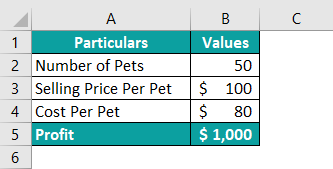Now, Mr. Rafal wants to know the profit scenario if he sells 40, 60, and 70 pets per day.

The steps used to perform What-If Analysis in excel are as follows:

Step 1: Under the Data tab, select Scenario Manager option from the Forecast group.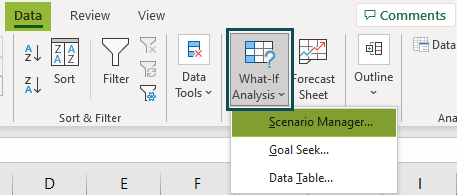Now, we can see Scenario Manager window as shown below.

Step 2: Let us type the scenario name as Less Likely in Scenario name: box and choose cell B2 in the Changing cells: section.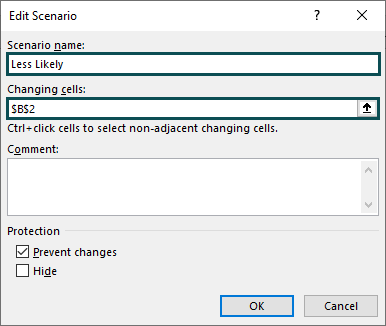Step 3: Click OK. The Scenario values window pops up. Change the cell value to 40.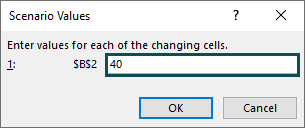Step 4: Click Add and create two other scenarios (for selling 60 and 70 pets per day) as Best Case and Less Realistic, respectively.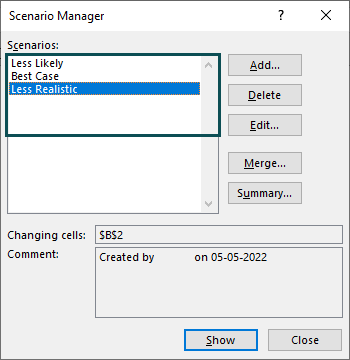Step 5: We have created three scenarios. Now, click on Summary to create a summary of all the scenarios. The Scenario Summary window pops up. Choose the Result cells: as B5 (Profit cell).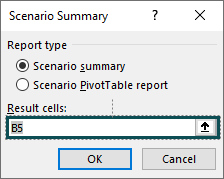Click OK, and we will have all the summary scenarios.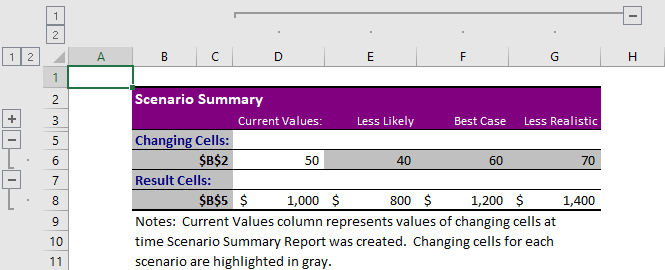With the obtained results, Mr. Rafal can now decide how many pets he should have daily to earn a better profit.

Where is What-If Analysis in Excel?

What-If Analysis in Excel is located under the Data tab in the Forecast group.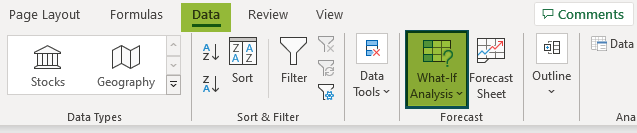We can select any of the three types under What-If Analysis drop-down list.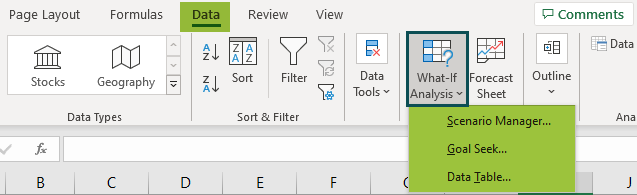What are the steps in What-If Analysis in Excel?

What-If Analysis comes with three different methods of analysis. They are:

1. Scenario Manager
2. Goal Seek
3. Data Table